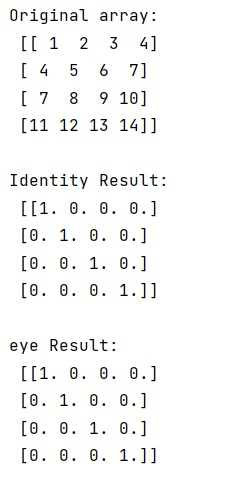# What are the advantages of using numpy.identity over numpy.eye?

Submitted by Pranit Sharma, on February 09, 2023

NumPy is an abbreviated form of Numerical Python. It is used for different types of scientific operations in python. Numpy is a vast library in python which is used for almost every kind of scientific or mathematical operation. It is itself an array which is a collection of various methods and functions for processing the arrays.

## Advantages of using numpy.identity() over numpy.eye()

The numpy.identity() returns the identity array of an array. The identity array is a square array with ones on the main diagonal.

On the other hand, numpy.eye returns a 2-D array with ones on the diagonal and zeros elsewhere.

The main difference is that with the numpy.eye() the diagonal can may be offset, whereas numpy.identity() only fills the main diagonal. Since the identity matrix is such a common construct in mathematics, it seems the main advantage of using numpy.identity() is for its name alone. So, the main advantage depends on the requirement. If we want an identity matrix, we can go for numpy.identity() right away or can call the numpy.eye() leaving the rest to defaults. But, if we need a 1's and 0's matrix of a particular shape/size or have control over the diagonal we can go for numpy.eye() method.

Let us understand with the help of an example,

## Python code to demonstrate the example of the advantages of using numpy.identity over numpy.eye

```# Import numpy
import numpy as np

# Creating a numpy array
arr =  np.array([[1, 2, 3, 4],
[4, 5, 6, 7],
[7, 8, 9, 10],
[11, 12, 13, 14]])

# Display original array
print("Original array:\n",arr,"\n")

# Using identity
res = np.identity(4)

# Display Identity Result
print("Identity Result:\n",res,"\n")

# Using eye
res = np.eye(4)

# Display eye Result
print("eye Result:\n",res,"\n")
```

Output: